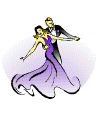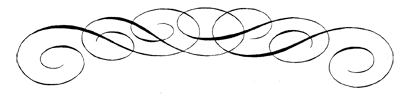## ROUND DANCING — CHOREOGRAPHED BALLROOM

### EDUCATIONAL ARTICLES

MAJOR SECTIONS: Figures | Articles | Links | Alph. Index | Search | Home

 BROWSE Figures in the Smooth Rhythms Foxtrot Quickstep Waltz Viennese Waltz International Tango American Tango Two Step Five Count One Step Polka Rhythm Figures in the Latin Rhythms Cha Cha Rumba Jive Single Swing West Coast Swing Lindy Hustle Bolero Slow Two Step Mambo Salsa Samba Argentine Tango Merengue Paso Doble Dance Articles Articles Home Dance Figures Dance Rhythms Lead and Follow Dance Styling Fred Astaire Album Other Sections Dance Links Music Clips For Each Rhythm Search Site/Web Sources Contact Me

## ROUNDALAB Phases 101

by Annette Woodruff

A number from 1 to 6, traditionally expressed as Roman numerals (I, II, III, IV, V, VI) but today also often expressed in plain Hindu-Arabic numerals (1, 2, 3, 4, 5, 6) is attributed to each figure. A phase I (1) figure would be the simplest, for instance, Walk, Run, Side Two Step, Box. A phase VI (6) figure, at the opposite end of the range, would be a complex figure like a Continuous Natural Top or a relatively simple figure the execution of which requires experience like a Ballerina Wheel.

All the rhythms are not represented in all the phases. Traditionally, two-step has been considered as the easiest rhythm, and was, for many years, the first rhythm that was introduced to newcomers. It is covered in phases I to III.

Waltz is the only rhythm that runs through all the phases, from I to VI.

A number of rhythms run from III to VI: bolero, cha cha, jive, foxtrot, mambo, merengue, quickstep, rumba, tango, and slow-two-step, even if some rhythms are currently lacking figures above a certain level.

Three rhythms are listed in phases IV to VI: paso-doble, samba, and Westcoast swing. From this you may surmise that round dancing considers these three rhythms harder than the rhythms that start at phase III, like cha cha and jive. You may also deduce that the rhythms that start at phase III are considered as more complex than two-step or the first level of waltz. This is a matter of regular controversy, especially since, in the recent years, teachers have started introducing phase III rhythms (like cha cha or rumba) to newcomers before phase I & II two-step.

Let’s look at how a dance is rated by its choreographer.

If all the figures within the dance are, for instance, phase II figures, the dance is rated phase II. If the dance contains mostly phase II figures but also contains one phase III figure, it is rated II+1. If it contains two phase III figures, it is rated II+2. But as soon as it contains three phase III figures, the dance is rated III.

Let’s suppose now that we have a waltz that contains only phase II figures except for one: a Telemark to Semi. The Telemark is a phase IV figure, so how do we rate this dance? If you spontaneously shouted “II+1!”, I do sympathize, but you missed the jackpot. The rating “II+1” would indicate a dance with phase II figures plus one phase III figure. The fact that it contains one phase IV figure makes it a phase III+1 dance, even if it does not contain a single phase III figure. Odd, isn’t it? Want something even odder? It is, in principle, possible to have a two-step rated IV+1, even though two-step is not represented in phase IV or V. It would suffice, for this, to end the dance with a Hinge (phase V), for instance “Ending: Box;; Back-2-Step; Hinge”. NOT A GOOD IDEA, I agree, but quite possible and even danceable.

A tentative addition to the system has recently been made for phase IV waltz and phase IV foxtrot. These two rhythms have, at phase IV, a large number of figures, i.e., about twice as many as the other rhythms normally have. The lists of these figures have therefore been split into IVa (easier figures/actions/movements) and IVb (more advanced figures/actions/movements). If all the figures, actions, and movements of a dance are from the IVa list or from a lower phase and there are no unphased figures/actions/movements in the dance, it can be rated as a IVa dance. There may not be any “+” to a IVa dance. As soon as there is a figure/action/movement from the IVb list, the dance needs to be either rated IVb or simply IV. This is also true if a dance uses multiple rhythms: if a dance is a foxtrot and jive, it cannot be rated IVa even if all the foxtrot figures in the dance are from the IVa list. It’s just a phase IV.

It only remains now to address the unphased figures, i.e., those that are NOT in the Roundalab manual NOR simply minor modifications of standard figures. A dance that contains phase III figures, plus one phase IV figure, plus one unphased figure would be rated III+1+1. A dance containing mostly phase III and IV figures as well as two unphased figures would be rated IV+0+2. And no, there is no limit to the number of unphased figures that you can have in a dance, so, in theory, you could have an IV+0+5 (NOT A GOOD IDEA either!). At phase VI, you can only add unphased figures, so there is no need to write VI+0+1, but just VI+1.

A few years ago Roundalab added a refinement to the phase rating. Recognizing that a phase V dance, for instance, could be very easy (containing only three phase V figures and no unusual feature), or very difficult (lots of transitions and picture figures for example), Roundalab encourages the choreographers to add to the phase rating, the appreciation EASY, AVERAGE, or DIFFICULT. “Easy” means that, if you are a dancer regularly dancing phase V, you can do this dance to cues. “Average” means that you would be able to dance it with one or two simple instructions. “Difficult” indicates that a special teach of the dance would be needed.

To end, here are a few examples of phase ratings and what they mean:
• II+1 = phase II figures plus one phase III figure
• II+2 = phase II figures plus two phase III figures
• II+0+1 = phase II figures plus one unphased figure.
• II+0+3 = phase II figures plus three unphased figures
• III+1 = phase II or III figures plus one phase IV figure
• III+2 = phase II or III figures plus two phase IV figures
• III+1+2 = phase II or III figures plus one phase IV figure & two unphased figures
• IV+1 = phase II, III, or IV figures plus one phase V figure
• IV+2 = phase II, III, or IV figures plus two phase V figures
• IV+0+4 = at least 3 phase IV figures plus four unphased figures
• IV+2+1 = phase II, III, or IV figures plus 2 phase V figures + 1 unphased figure
• V+1 = phase II, III, IV, or V figures plus one phase VI figure
• V+2 = phase II, III, IV, or V figures plus two phase VI figures
• V+0+1 = at least 3 phase V figures plus one unphased figure
• VI+2 = at least 3 phase VI figures plus 2 unphased figures

If you've got all that, thank you for your patience and you are now a Phase Expert.

From RoundMusic posts, Nov & Dec 2021, and reprinted in the Dixie Round Dance Council (DRDC) Newsletter, June 2022.Alphabetical Index to Figures and Technique Dance Figures Dance Articles Dance Search Dance Links Dance Home